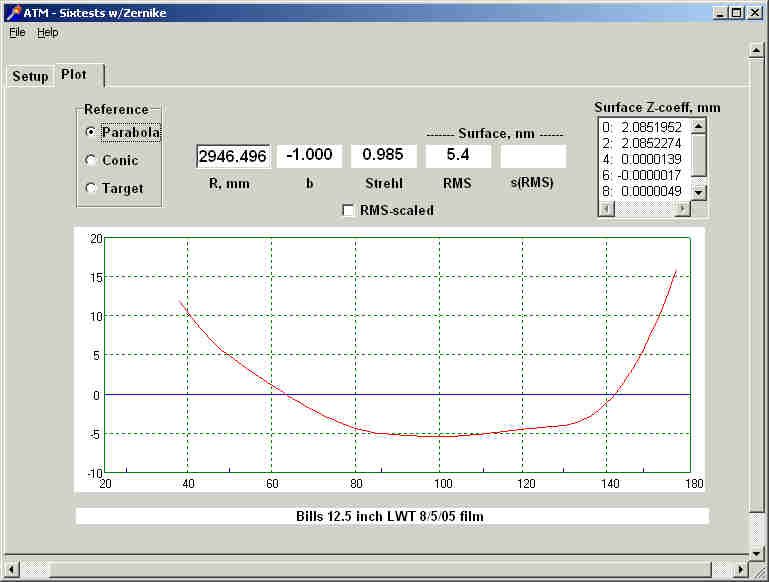## Film Slit - Testing Telescope Mirrors - Bill Thomas(send comments to bthomas32000@yahoo.com)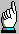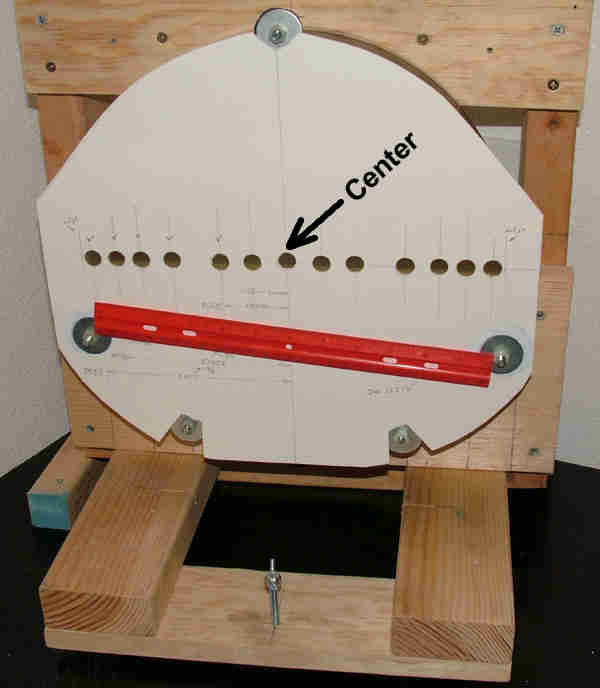The mask is computer scanned for the purpose of accurately measuring the hole pair spacing. The center hole is used to align the tester with the optical axis. This is done using an eyepiece. Sharpie dot the center of the mirror to align the mask. Use a level to align the holes

The reflected slit (0.003" tester slit) images from the holes are recorded on film, which is located in front or behind the Radius of Curvature - ROC. My exposures are done aft of ROC by about 1.250". The ray cross each other in back of ROC and the film plane must be some amount aft of where the two outer zones intersect:

l = (h3n-1 - h3n)/(2R(hn-1 - hn)) + some amount
The processed film is then computer scanned and the distance, Ym between the slit pair's measured in PhotoShop.

Next Sixtests.exe is fired up in Caustic Fixed Source Lateral Wire Test mode with zone inputs of -Ym/2 (the half below the optical axis).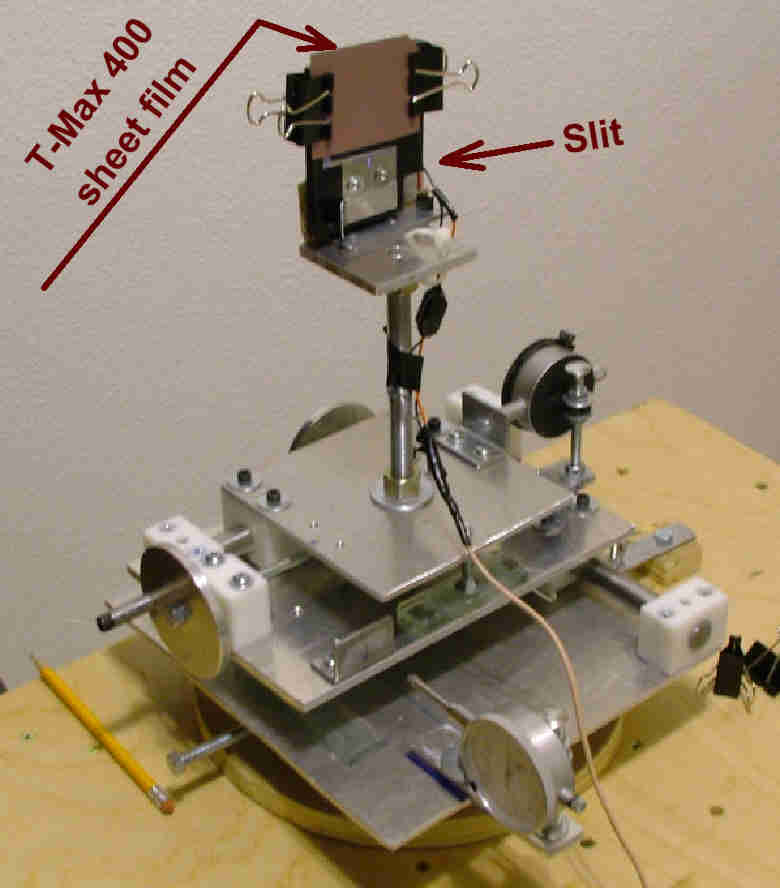The film plane should be at right angles to the optical axis. Replace the film with a plastic mirror, and then project a laser through a hole in the plastic mirror to the center of the mirror under test. Adjust the film plane until the second reflection returns to the center of the mirror under test. Film Slit tester doesn't require as an elaborate tester as the one pictured above. This tester was constructed for LWT. But due to environmental variations e.g., temperature it was impossible to obtain meaningful data. The Film Slit test solves this problem in that all slit measurements are recorded at the same moment in time.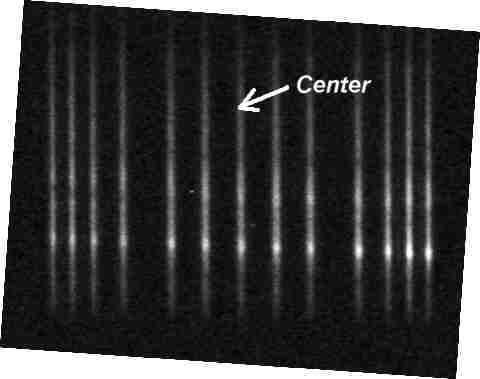Slit images (Tmax 400, 30 second exposure. Developed 7 minutes - HC110 Dilution B - 7 parts water to one part stock). The center ray is used to align the tester to the optical axis. Also check, to be sure that the center slit image is very nearly equally between the slit pairs. The full size, undistorted (953 K byte TIFF file) version of this image is available here.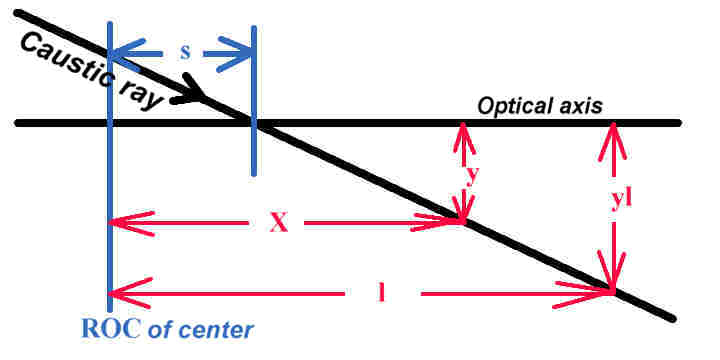### Moving source:  yl = (l - s)h/R;   Sagitta, s = l - RYm/2h Fixed source:   yl = 2(l - s)h/R;   Sagitta, s = l - RYm/4h

Moving source:
R = ROC
sagitta, s = h2/(2R); //ignores term +h4/(4R3)
Caustic:
X = 3h2/(2R) or 3s
Y = 2h3/R2 or 4hs/R
y = Y/2 or 2hs/R
Similar triangles
yl = (l - s)y/(X - s)
yl = (l - s)2sh/R/(3s-s)
yl = (l - s)2sh/(2sR)
yl = (l - s)h/R

sagitta, s = l - Ryl/h
For fixed source double yl

The Film Slit Ym's are fixed source measurements. That is yl = Ym/4 and Sagitta, s = l - RYm/(4h)

Example (Film_Slit.xls. Besides Excel, there is a free spreadsheet, OpenOffice at http://www.openoffice.org/) is for a 12.5" f 4.7 mirror.

Required inputs:
Optical Diameter of the mirror B2
The scanned DPI J3
The scanned films measured slits Ym J6:K11
In Photoshop:
Rotate the image (my scanner is most accurate in the direction of scanner travel and in the center):
Enlarge the image greatly. Using the "measure tool" draw a line through the center of a slit image.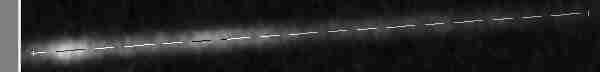Image->Rotate Canvas->Arbitrary dialog box opens "Rotate Canvas" click OK (the angle is already there to level the slits).
On the "Info pallet" adjust the the measure tool "H" so as to be zero. Then drag the measure tool to the center of each slit image and read off the "Y", proceeding from the top slit to the bottom slit. The spreadsheet does the differences to get the pixel separation and then divides by the the scanned DPI J3 e.g., 6400 pixels/inch.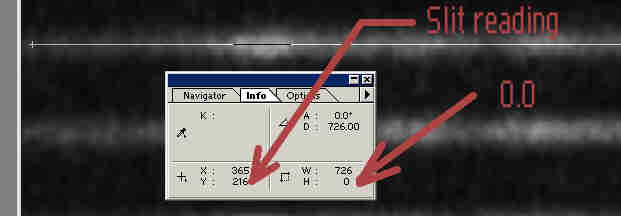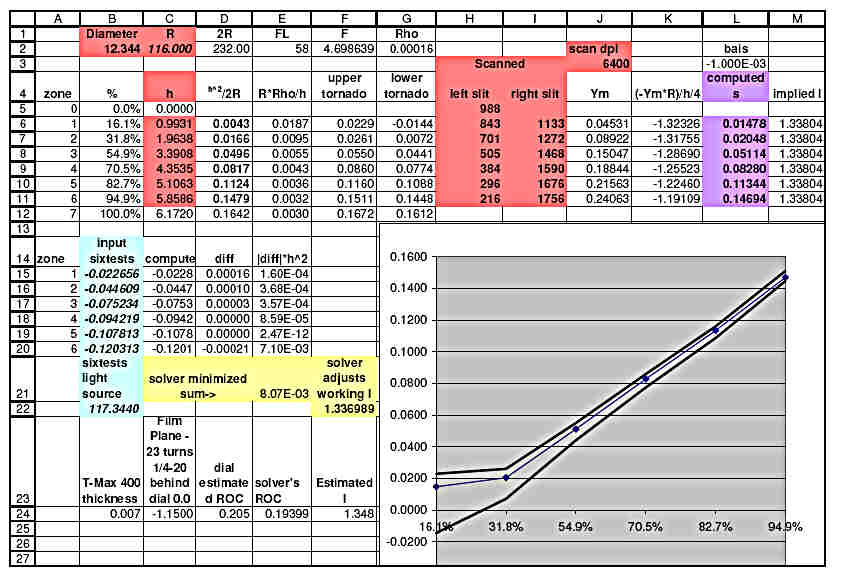At first glance, computed s should be s = l - Ryl/h. However, l depends on determining the location of ROC (center of the mirror) which can be difficult, which in turn makes l problematic. The approach (Glen Youman's idea http://www.astrophotos.net/) taken instead, is to base the computed s on the 94.9% zone as follows:

Ryl/h for each zone, is added to the computed s (h2/(2R) of the 94.9% zone minus Ryl/h of the 94.9% zone (\$D\$11+K6 -\$K\$11, …………, \$D\$11+K11 -\$K\$11). This normalizes all zone to the 94.9% zone. Notice that l is computed to be 1.33804" (M6-M11). The value of l determined from the measured ROC was 1.3480" (E24). A 0.010" measurement error of the ROC.

Besides the -yl/2 input (B15 - B20), Sixtests.exe also needs an accurate l This is accomplished with the Excel's solver (Tools->solver). Cells D15 - D20 contain the expected -yl/2 as a function of working l (F24). Solver changes working l to minimize the sum of the weighted (h2) differences (-yl/2 - computed -yl/2) in E21. working l and light source along with -yl/2 (B15 - B20) are then the inputs to Sixtests.exe. Notice that the Implied l 1.3380" and Working l 1.336988" are in very close agreement, differing by only 0.001".

```Bills 12.5 inch LWT 8/5/05 film
12.344	3	Mirror, obstruction diameters (mm)
caustic
117.3440 Source distance (0=moving source)
y, mm	X, mm	Y, mm
0.99305 1.336988 -0.022656
1.96375 1.336988 -0.044609
3.39078 1.336988 -0.075234
4.35352 1.336988 -0.094219
5.10625 1.336988 -0.107812
5.85859 1.336988 -0.120312
*
-1.000	0.0 Conic targets:  b, R (mm)
0.0000	Measurement std deviation, mm